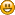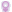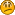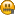###Author Topic: Quadratics and Trigonometry Problems!  (Read 1354 times)

####whale95

• Newbie
•• Posts: 42
• Reputation: 65535« on: December 28, 2011, 07:36:32 pm »
Note =  means to the power of 2

Q) The equation of the curve is y = 3cos2x. The equation of a line is x+2y=? (pi) . On the same Diagram, sketch the curve and the line for 0<x<? .
Find the first 3 terms in the expansion of (2-x) in ascending power of x.
I did the graph but didnt know how to do the line! :S

Q)2cos? = 1/tan?          0<?<2?
I did upto cos?(2sin?-1)=0
so cos?=0  so 0
and 2sin?-1=0 so ?/6 and 5?/6
so now I have 3 answers which are in bold! how did ?/2 and 3?/2 come??

Q)sinx=3/5 and angle is obtuse. Find tanx and cosx

..........

Identities:
Q) (1+tan?)cos? = 1

Q) sin?/1-cos? = 1+cos?

Q) 1-tan?/1+tan? = 1-2sin?

.........

Q) Find the nature of the root? 2x - ax + 2a = 0

Q) x - 2x + 1 = p(x-3) has equal roots find P??

i did b - 4ac
(-2-p)(-2-p)-4(1)(1+3p) = 0

Thank you in advance!####~ Miss Relina ~

• SF Senior Citizen
••• Posts: 611
• Reputation: 65535
• Gender:• Every rose has a thorn##### Re: Quadratics and Trigonometry Problems!
« Reply #1 on: December 29, 2011, 07:40:35 am »
Mr.Astarmathandphysics anwered them there  :they are named  out and out2

post your doubts there and he will answer you so that to avoid confusion~~~Yarab everything turns alright at the end ~~~
-----------Ameeeeeeeeeeen------------

####SenjaginHQ

• Newbie
•• Posts: 7
• Reputation: 0